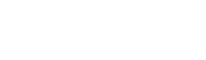# On surrogate models using PINNs (Physics-Informed Neural Networks)

スポンサーリンク

Hello, I am Yuki (@engieerblog_Yu), who is doing research using surrogate models in the Visualization Laboratory.

スポンサーリンク

## What is PINNs?

Physics-Informed Neural Networks (PINNs) is a method of incorporating physics constraints into neural networks to allow them to make more accurate predictions.

Such methods are very useful in solving physics problems.

For example, when predicting temperature or flow distributions, a normal neural network can make predictions by training the model through input and output data.

However, with this method, if the input and output data do not match, accurate predictions may not be possible.

By using PINN, accurate forecasts can be made even when the input and output data do not match, because the model can be trained using basic physics-based equations.

This allows for more accurate predictions.

PINN is also useful in solving complex physics problems because of its high computational speed compared to ordinary neural networks.

## Why PINNs are used in surrogate models

PINNs are used in surrogate models because they are computationally faster than simulations.

In a surrogate model, the governing equations used in the simulation are estimated by a neural network using inputs and outputs.

The governing equations contain physical constraints, which means that surrogate models and PINNs are a good match.

The physical constraints are incorporated into the loss function of the neural network.

The neural network updates the weights so that the error due to the loss function is small, so the physical constraints can also be factored into the optimization.

## Summary

PINNs (Physics-Informed Neural Networks) incorporate physics constraints into neural networks.

Physical constraints are incorporated into the loss function of the neural network, and physical constraints can also be taken into account in the optimization.